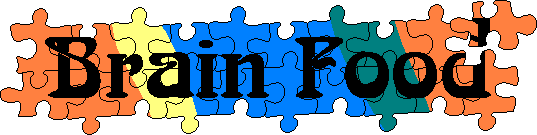Main      Site Guide### Word Ends

In each of these puzzles, a word starts and ends with the same letter. Can you figure out what the word is?

_omi_

Solution

_ido_

Solution

_ene_

Solution

_ende_

Solution

_abe_

Solution

_allof_

Solution

_erami_

Solution

_hwar_

Solution

_ogna_

Solution

_uzza_

Solution

_arach_

Solution

_umul_

Solution

_eart_

Solution

_icku_

Solution

_ios_

Solution

Solution

_zale_

Solution

_asba_

Solution

_rac_

Solution

_rai_

Solution

_ras_

Solution

_oca_

Solution

_pne_

Solution

_infol_

Solution

_arge_

Solution